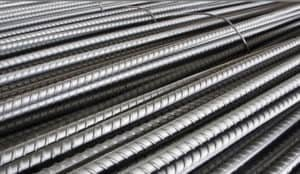## Calculation Of Unit Weight Of Steel Bars:After estimating it is very important to know the unit weight of steel bars because, we estimate as 100 meter 20mm ø bar or 100 feet 16mm ø bar, etc (ø is the symbol of diameter).

But steel bar suppliers will not understand this notation, they measure the steel bars in weight. So we have to order them in kg or quintal or ton. In this article, we will discuss how to calculate unit weight of steel bars of different diameter.

The formula is W = D²L/162

Where

W = Weight of steel bars.

D = Diameter of steel bars in mm.

L = Length of bars in meter.

Example 1: Calculate the weight of 60 meters long 12 mm ø bar.

Here, D = 12 mm.

L= 60 m.

We know that, W = D²L/162

W = 12² x 60/162 = 53 kg

Weight of 60 m 12mm ø bar is 53 kg.

Let’s look for another example.

Example 2: Calculate the weight of 100 m 16 mm ø bar.

Here, D = 16 mm.

L = 100 m.

W = 16² x 100/162 = 158 kg.

If we put 1 meter length for each diameter of steel bar in the formula then we will get the unit weight.

• 10mm ø bar = 10² x 1/162 = 0.617 kg/m
• 12mm ø bar = 12² x 1/162 = 0.888 kg/m
• 16mm ø bar = 16² x 1/162 = 1.580 kg/m
• 20mm ø bar = 20² x 1/162 = 2.469 kg/m

If we multiply the length of bars with this unit weight we will get the total weight of steel bars.

For example, total weight of 1000 meter long 20mm ø steel bar is,

1000 x 2.469 = 2469 kg.

Using the same method we can calculate the unit weight of different steel bars.

Here I have calculated in meter but we can also calculate in foot. To calculate in foot we have to use the following formula:

W= D²L/533

Where D = Diameter of bars in mm.

L = Length of bars in foot.

##### Also Read – How To Calculate Cement, Sand Aggregates For Concrete.

1. how do you obtain the formula?

2. This is very helpful for those in management position who are oblige to perform quantification in terms of construction schedule planning. I want and kindly request full time subscription in your daily newsletter.

3. Tnx for information calculate unit of steel bars and I hope u will gave me some information of civil work next tme
Pls keep it up

Thanking you sir

in L=100m, and
in question L=60m,
some mistake or confusion are there

• Thanks for informing about the mistake. We have updated it as well.

5. Steel Wight farmul

6. Thank you very much for putting us through.
But we will like the pdf copy.

7. Thanks for informition

8. Really thank you dear sir

9. You said about L-lenth, W-weight and D-Diametre what about 162? why only 162? not other number? Can you explain it?

10. we dont know what is 162 or where this 162 came from? If You know, please explain what is it.